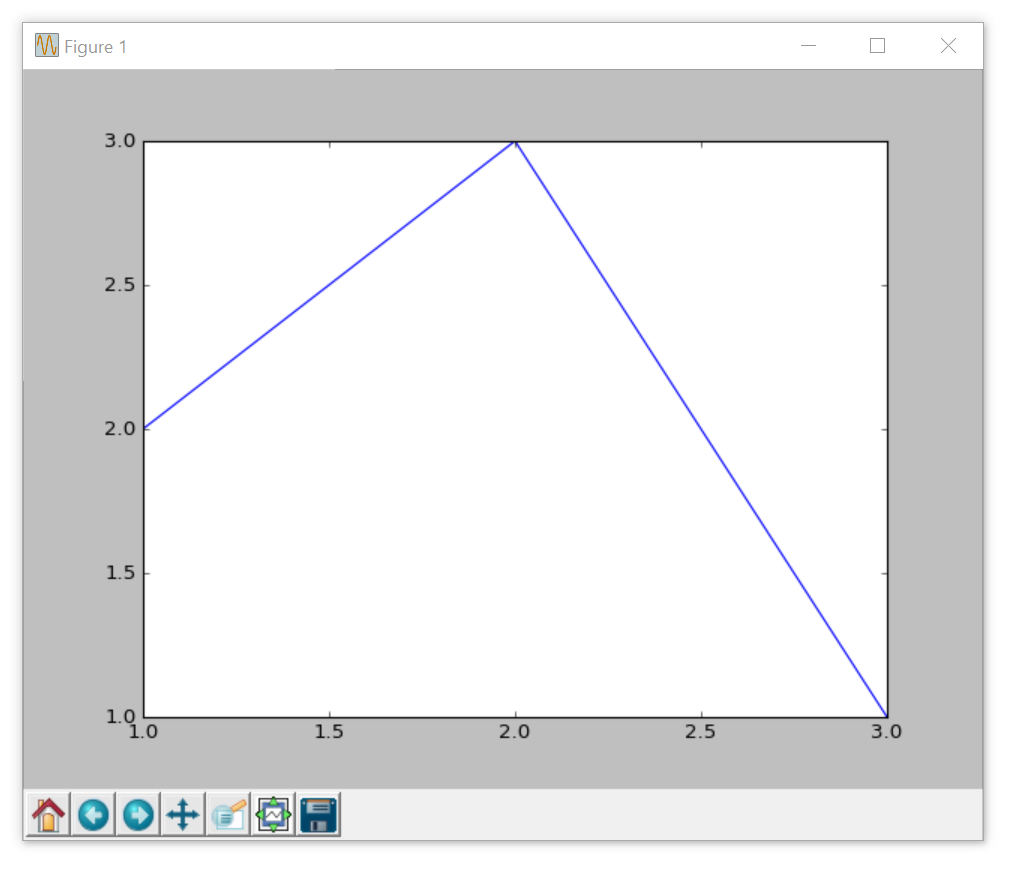## Args and Kwargs

Welcome to part 25 of the intermediate Python programming tutorial series. In this tutorial, we're going to cover `*args` and `**kwargs`.

The idea behind `*args` and `**kwargs` is that there may be times when you have a function and you want to be able to handle an unknown number of arguments. The `*args` will handle for any number of parameters, and `**kwargs` will handle for any number of keyword arguments (hence kwargs). Let's see some examples. Let's say you've got a blog with some posts saved to variables. Something like:

```blog_1 = 'I am so awesome'
blog_2 = 'Cars are cool.'
blog_3 = 'Aww look at my cat.'```

Now, you can have a function that iterates through them and just prints them:

```def blog_posts(*args):
for post in args:
print(post)```

It's very similar to if you were to pass a list as a parameter, then iterated through that list. We can do:

`blog_posts(blog_1)`

Output:

`I am so awesome`

We can also do:

`blog_posts(blog_1,blog_2,blog_3)`

Output:

```I am so awesome
Cars are cool.
Aww look at my cat.```

We can also use `*args` with regular parameters:

```def blog_posts_2(regular_arg, *args):
print(regular_arg)
for post in args:
print(post)

blog_posts_2('My blogs:',blog_1,blog_2,blog_3)```

Output:

```My blogs:
I am so awesome
Cars are cool.
Aww look at my cat.```

Now, there will be many times where you want args that are still assigned to some sort of name, which is where keyword arguments, `**kwargs` come in! Where `*args` are like a list, `**kwargs` are like a dictionary.

```def blog_posts_3(**kwargs):
for title, post in kwargs.items():
print(title,post)

blog_posts_3(blog_1 = 'I am so awesome',
blog_2 = 'Cars are cool.',
blog_3 = 'Aww look at my cat.')```

Output:

```blog_2 Cars are cool.
blog_1 I am so awesome
blog_3 Aww look at my cat.```

Interestingly, you can also use `*args` when passing arguments to a function, even when that function wasn't built to accept an unlimited number of arguments. For example, let's say you've got a function that graphs, but for now we can just print:

```def graph_operation(x, y):
print('function that graphs {} and {}'.format(x, y))```

Rather than passing x and y explicitly, we can pass it as args, like:

```def graph_operation(x, y):
print('function that graphs {} and {}'.format(x, y))

x = [1,2,3]
y = [2,3,1]
graph_me = [x,y]
graph_operation(*graph_me)```

Output:

`function that graphs [1, 2, 3] and [2, 3, 1]`

If you have matplotlib installed, we can actually graph it:

```import matplotlib.pyplot as plt

def graph_operation(x, y):
print('function that graphs {} and {}'.format(x, y))
plt.plot(x,y)
plt.show()

x = [1,2,3]
y = [2,3,1]
graph_me = [x,y]
graph_operation(*graph_me)```

Output:The next tutorial:• Intermediate Python Programming introduction

• String Concatenation and Formatting Intermediate Python Tutorial part 2

• Argparse for CLI Intermediate Python Tutorial part 3

• List comprehension and generator expressions Intermediate Python Tutorial part 4

• More on list comprehension and generators Intermediate Python Tutorial part 5

• Timeit Module Intermediate Python Tutorial part 6

• Enumerate Intermediate Python Tutorial part 7

• Python's Zip function

• More on Generators with Python

• Multiprocessing with Python intro

• Getting Values from Multiprocessing Processes

• Multiprocessing Spider Example

• Introduction to Object Oriented Programming

• Creating Environment for our Object with PyGame

• Many Blobs - Object Oriented Programming

• Blob Class and Modularity - Object Oriented Programming

• Inheritance - Object Oriented Programming

• Decorators in Python Tutorial